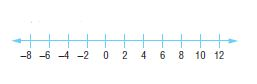Homework Explained - Math Practice 101Dear guest, you are not a registered member. As a guest, you only have read-only access to our books, tests and other practice materials.

As a registered member you can:

Registration is free and doesn't require any type of payment information. Click here to Register.
Go to page:
Chapter 3: Integers; Lesson 1: Integers and Absolute Value

### Guided Practice

Write an integer for each situation.

• Question 1

a deposit of $16 • $
• Question 2

a loss of 11 yards

•  yards
• Question 3

6°F below zero

•  $$^o\text{F}$$

Evaluate each expression.

•  $$|-9| =$$
•  $$|18| - |-10| =$$
•  $$|-11| - |-6| =$$
• Question 7

Graph the set of integers {11, -5, -8} on a number line.• Question 8

Building on the Essential Question Why is the absolute value of a number positive? Explain your reasoning.

Yes, email page to my online tutor. (if you didn't add a tutor yet, you can add one here)